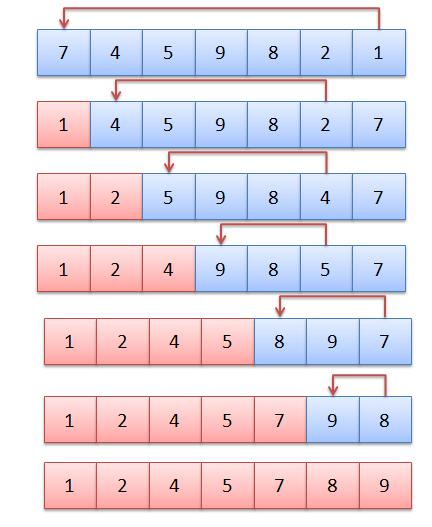# Selection Sort

Selection Sort

Selection sort is a simple sorting algorithm. This sorting algorithm is an in-place comparison-based algorithm in which the list is divided into two parts, the sorted part at the left end and the unsorted part at the right end. Initially, the sorted part is empty and the unsorted part is the entire list.Selection Sort

The smallest element is selected from the unsorted array and swapped with the leftmost element, and that element becomes a part of the sorted array. This process continues moving unsorted array boundary by one element to the right.

This algorithm is not suitable for large data sets as its average and worst case complexities are of Ο(n2), where n is the number of items.

How Selection Sort Works, refer link for more details

#include<iostream>
using namespace std;

class Selection
{
public:
void Selection_Sort(int a[],int n);
void Display_array(int a[], int n);
};

void Selection::Selection_Sort(int a[], int n)
{
int Amin=0;
for(int i=0;i<n-1;i++)
{
Amin=i;
for(int j=i+1;j<n;j++)
{
if(a[j]<a[Amin])
Amin=j;
}
int temp = a[i];
a[i]= a[Amin];
a[Amin]= temp;

}
}
void Selection::Display_array(int a[], int n)
{
cout<<"\n\n Sorted elements are :-"<<endl;
for(int i=0;i<n;i++)
{
cout<<a[i]<<" ";
}
cout<<"\n\n";
}
int main()
{
int a,n;
cout<<"\n\nenter no of elements:- ";
cin>>n;

cout<<"\nenter array elements:-"<<endl;
for(int i=0;i<n;i++)
{
cin>>a[i];
cout<<endl;
}
Selection obj;
obj.Selection_Sort(a,n);
obj.Display_array(a,n);

}